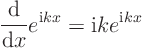# QM explanation of electron conduction in crystal

Suppose that a static electric field E is applied on a conductive crystal. Then, how quantum mechanics describes the electron movements in the crystal?

I have been trying to find an explanation, but have not found any.

As I understand it, we could treat the E field as a time-dependent perturbation, starting at t=0. But after sufficient time E would not excite any electron to any higher energy level.

However, if the electron's wavefunction is a combination of more than one Bloch functions/states, then the perturbation of E can excite the electrons to different ones of these Bloch states, which could be an origin of electron conduction in crystal.

One question, however, is that such excitation is "symmetrical", that is, it is not directional as the E field is.

Is there any problem with these considerations?

I don't know why you would think it is symmetrical. For actual conduction in crystal we must consider the lattice in more ways than it just being a periodic potential. Otherwise we might find that an electron in an E field just accelerates forever. A crude approach is to just use "relaxation time" in a semi-classical model to account for the lattice, and then consider the motions in the periodic potential with applied external field to describe what happens in between thermalizing collisions with the lattice.

For this let's introduce a ##\boldsymbol{\kappa}## operator, so that if ##\mid n,\,\mathbf{k}\rangle## is a Bloch wave state ##\boldsymbol{\kappa}\mid n,\,\mathbf{k}\rangle = \mathbf{k} \mid n,\,\mathbf{k}\rangle##

In the Heisenburg picture

##\dot{\boldsymbol{\kappa}} = \frac{i}{\hbar} \left[H, \boldsymbol{\kappa}\right]##
We have ##H = H_{crystal} + H_{external}## where ##H_{crystal}## includes kinetic term plus the periodic potential, and ##H_{external}## represents the applied field. Because the Bloch wave states were already eigenvectors of ##H_{crystal}## We are left with
$$\dot{\boldsymbol{\kappa}} = \frac{i}{\hbar} \left[H_{external}, \boldsymbol{\kappa}\right]$$

We can introduce a semi-classical wave packet which has both a central position x and a central bloch wavevector k. We can create a semiclassical framework such that
$$\dot{\mathbf{k}} = \frac{i}{\hbar}\left(-e \mathbf{E} \right)$$
$$\dot{\mathbf{x}} = \frac{1}{\hbar}\nabla \epsilon_n(\mathbf{k})$$

Where ##\epsilon_n(\mathbf{k}) ## represents the energies in the n-th band. This topic is covered in Ch. 12 of Ashcroft and Mermin. This may not be the final word on the subject but I hope it helps.

I don't know why you would think it is symmetrical. For actual conduction in crystal we must consider the lattice in more ways than it just being a periodic potential. Otherwise we might find that an electron in an E field just accelerates forever. A crude approach is to just use "relaxation time" in a semi-classical model to account for the lattice, and then consider the motions in the periodic potential with applied external field to describe what happens in between thermalizing collisions with the lattice.

For this let's introduce a ##\boldsymbol{\kappa}## operator, so that if ##\mid n,\,\mathbf{k}\rangle## is a Bloch wave state ##\boldsymbol{\kappa}\mid n,\,\mathbf{k}\rangle = \mathbf{k} \mid n,\,\mathbf{k}\rangle##

In the Heisenburg picture

##\dot{\boldsymbol{\kappa}} = \frac{i}{\hbar} \left[H, \boldsymbol{\kappa}\right]##
We have ##H = H_{crystal} + H_{external}## where ##H_{crystal}## includes kinetic term plus the periodic potential, and ##H_{external}## represents the applied field. Because the Bloch wave states were already eigenvectors of ##H_{crystal}## We are left with
$$\dot{\boldsymbol{\kappa}} = \frac{i}{\hbar} \left[H_{external}, \boldsymbol{\kappa}\right]$$

We can introduce a semi-classical wave packet which has both a central position x and a central bloch wavevector k. We can create a semiclassical framework such that
$$\dot{\mathbf{k}} = \frac{i}{\hbar}\left(-e \mathbf{E} \right)$$
$$\dot{\mathbf{x}} = \frac{1}{\hbar}\nabla \epsilon_n(\mathbf{k})$$

Where ##\epsilon_n(\mathbf{k}) ## represents the energies in the n-th band. This topic is covered in Ch. 12 of Ashcroft and Mermin. This may not be the final word on the subject but I hope it helps.

Semi-classical wave packet, or localization of wave function (WF) of electron, works well as basis for explaining electron transportation in semi-conductors. But it seems failed in explaining superconditivity and normal conductivity in a single crystal.

In addition, it is doubtful whether the WF of an electron in a single crystal can be localized; the WF would be a summation of discrete Bloch functions. Can any summation of a set of (a limited number of) discrete periodic functions be local? This question seems partly taken care of in the textbook material at:
http://www.eng.fsu.edu/~dommelen/quantum/style_a/packets.html

where it is said in its discussion of wave packet:

"If the crystal is infinite in size, the wave numbercan take any value. (For a crystal in a finite-size periodic box as studied in chapter 6.22, the values ofare discrete. However, this subsection will assume an infinite crystal.)"

Does it indicate that a wave packet cannot be realized in a true (finite) single crystal?

DrDu